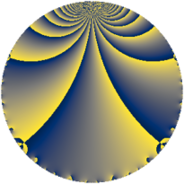# Properties

 Label 3200.2.f.dLevel $3200$ Weight $2$ Character orbit 3200.f Analytic conductor $25.552$ Analytic rank $0$ Dimension $2$ CM discriminant -4 Inner twists $4$

# Related objects

## Newspace parameters

 Level: $$N$$ $$=$$ $$3200 = 2^{7} \cdot 5^{2}$$ Weight: $$k$$ $$=$$ $$2$$ Character orbit: $$[\chi]$$ $$=$$ 3200.f (of order $$2$$, degree $$1$$, not minimal)

## Newform invariants

 Self dual: no Analytic conductor: $$25.5521286468$$ Analytic rank: $$0$$ Dimension: $$2$$ Coefficient field: $$\Q(\sqrt{-1})$$ Defining polynomial: $$x^{2} + 1$$ Coefficient ring: $$\Z[a_1, \ldots, a_{17}]$$ Coefficient ring index: $$2$$ Twist minimal: no (minimal twist has level 128) Sato-Tate group: $\mathrm{U}(1)[D_{2}]$

## $q$-expansion

Coefficients of the $$q$$-expansion are expressed in terms of $$i = \sqrt{-1}$$. We also show the integral $$q$$-expansion of the trace form.

 $$f(q)$$ $$=$$ $$q -3 q^{9} +O(q^{10})$$ $$q -3 q^{9} + 4 q^{13} + 2 i q^{17} + 4 i q^{29} -12 q^{37} + 10 q^{41} + 7 q^{49} -4 q^{53} + 12 i q^{61} + 6 i q^{73} + 9 q^{81} + 10 q^{89} + 18 i q^{97} +O(q^{100})$$ $$\operatorname{Tr}(f)(q)$$ $$=$$ $$2q - 6q^{9} + O(q^{10})$$ $$2q - 6q^{9} + 8q^{13} - 24q^{37} + 20q^{41} + 14q^{49} - 8q^{53} + 18q^{81} + 20q^{89} + O(q^{100})$$

## Character values

We give the values of $$\chi$$ on generators for $$\left(\mathbb{Z}/3200\mathbb{Z}\right)^\times$$.

 $$n$$ $$901$$ $$1151$$ $$2177$$ $$\chi(n)$$ $$-1$$ $$1$$ $$-1$$

## Embeddings

For each embedding $$\iota_m$$ of the coefficient field, the values $$\iota_m(a_n)$$ are shown below.

For more information on an embedded modular form you can click on its label.

Label $$\iota_m(\nu)$$ $$a_{2}$$ $$a_{3}$$ $$a_{4}$$ $$a_{5}$$ $$a_{6}$$ $$a_{7}$$ $$a_{8}$$ $$a_{9}$$ $$a_{10}$$
449.1
 − 1.00000i 1.00000i
0 0 0 0 0 0 0 −3.00000 0
449.2 0 0 0 0 0 0 0 −3.00000 0
 $$n$$: e.g. 2-40 or 990-1000 Significant digits: Format: Complex embeddings Normalized embeddings Satake parameters Satake angles

## Inner twists

Char Parity Ord Mult Type
1.a even 1 1 trivial
4.b odd 2 1 CM by $$\Q(\sqrt{-1})$$
40.e odd 2 1 inner
40.f even 2 1 inner

## Twists

By twisting character orbit
Char Parity Ord Mult Type Twist Min Dim
1.a even 1 1 trivial 3200.2.f.d 2
4.b odd 2 1 CM 3200.2.f.d 2
5.b even 2 1 3200.2.f.c 2
5.c odd 4 1 128.2.b.b 2
5.c odd 4 1 3200.2.d.e 2
8.b even 2 1 3200.2.f.c 2
8.d odd 2 1 3200.2.f.c 2
15.e even 4 1 1152.2.d.d 2
20.d odd 2 1 3200.2.f.c 2
20.e even 4 1 128.2.b.b 2
20.e even 4 1 3200.2.d.e 2
40.e odd 2 1 inner 3200.2.f.d 2
40.f even 2 1 inner 3200.2.f.d 2
40.i odd 4 1 128.2.b.b 2
40.i odd 4 1 3200.2.d.e 2
40.k even 4 1 128.2.b.b 2
40.k even 4 1 3200.2.d.e 2
60.l odd 4 1 1152.2.d.d 2
80.i odd 4 1 256.2.a.b 1
80.i odd 4 1 6400.2.a.l 1
80.j even 4 1 256.2.a.c 1
80.j even 4 1 6400.2.a.m 1
80.s even 4 1 256.2.a.b 1
80.s even 4 1 6400.2.a.l 1
80.t odd 4 1 256.2.a.c 1
80.t odd 4 1 6400.2.a.m 1
120.q odd 4 1 1152.2.d.d 2
120.w even 4 1 1152.2.d.d 2
160.u even 8 2 1024.2.e.k 4
160.v odd 8 2 1024.2.e.k 4
160.ba even 8 2 1024.2.e.k 4
160.bb odd 8 2 1024.2.e.k 4
240.z odd 4 1 2304.2.a.p 1
240.bb even 4 1 2304.2.a.p 1
240.bd odd 4 1 2304.2.a.a 1
240.bf even 4 1 2304.2.a.a 1

By twisted newform orbit
Twist Min Dim Char Parity Ord Mult Type
128.2.b.b 2 5.c odd 4 1
128.2.b.b 2 20.e even 4 1
128.2.b.b 2 40.i odd 4 1
128.2.b.b 2 40.k even 4 1
256.2.a.b 1 80.i odd 4 1
256.2.a.b 1 80.s even 4 1
256.2.a.c 1 80.j even 4 1
256.2.a.c 1 80.t odd 4 1
1024.2.e.k 4 160.u even 8 2
1024.2.e.k 4 160.v odd 8 2
1024.2.e.k 4 160.ba even 8 2
1024.2.e.k 4 160.bb odd 8 2
1152.2.d.d 2 15.e even 4 1
1152.2.d.d 2 60.l odd 4 1
1152.2.d.d 2 120.q odd 4 1
1152.2.d.d 2 120.w even 4 1
2304.2.a.a 1 240.bd odd 4 1
2304.2.a.a 1 240.bf even 4 1
2304.2.a.p 1 240.z odd 4 1
2304.2.a.p 1 240.bb even 4 1
3200.2.d.e 2 5.c odd 4 1
3200.2.d.e 2 20.e even 4 1
3200.2.d.e 2 40.i odd 4 1
3200.2.d.e 2 40.k even 4 1
3200.2.f.c 2 5.b even 2 1
3200.2.f.c 2 8.b even 2 1
3200.2.f.c 2 8.d odd 2 1
3200.2.f.c 2 20.d odd 2 1
3200.2.f.d 2 1.a even 1 1 trivial
3200.2.f.d 2 4.b odd 2 1 CM
3200.2.f.d 2 40.e odd 2 1 inner
3200.2.f.d 2 40.f even 2 1 inner
6400.2.a.l 1 80.i odd 4 1
6400.2.a.l 1 80.s even 4 1
6400.2.a.m 1 80.j even 4 1
6400.2.a.m 1 80.t odd 4 1

## Hecke kernels

This newform subspace can be constructed as the intersection of the kernels of the following linear operators acting on $$S_{2}^{\mathrm{new}}(3200, [\chi])$$:

 $$T_{3}$$ $$T_{7}$$ $$T_{11}$$ $$T_{13} - 4$$ $$T_{31}$$

## Hecke characteristic polynomials

$p$ $F_p(T)$
$2$ $$T^{2}$$
$3$ $$T^{2}$$
$5$ $$T^{2}$$
$7$ $$T^{2}$$
$11$ $$T^{2}$$
$13$ $$( -4 + T )^{2}$$
$17$ $$4 + T^{2}$$
$19$ $$T^{2}$$
$23$ $$T^{2}$$
$29$ $$16 + T^{2}$$
$31$ $$T^{2}$$
$37$ $$( 12 + T )^{2}$$
$41$ $$( -10 + T )^{2}$$
$43$ $$T^{2}$$
$47$ $$T^{2}$$
$53$ $$( 4 + T )^{2}$$
$59$ $$T^{2}$$
$61$ $$144 + T^{2}$$
$67$ $$T^{2}$$
$71$ $$T^{2}$$
$73$ $$36 + T^{2}$$
$79$ $$T^{2}$$
$83$ $$T^{2}$$
$89$ $$( -10 + T )^{2}$$
$97$ $$324 + T^{2}$$